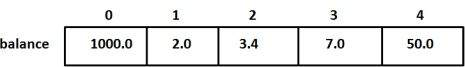# C++ Arrays

C++ provides a data structure, the array, which stores a fixed-size sequential collection of elements of the same type. An array is used to store a collection of data, but it is often more useful to think of an array as a collection of variables of the same type.
Instead of declaring individual variables, such as number0, number1, ..., and number99, you declare one array variable such as numbers and use numbers, numbers, and ..., numbers to represent individual variables. A specific element in an array is accessed by an index.
All arrays consist of contiguous memory locations. The lowest address corresponds to the first element and the highest address to the last element.

## Declaring Arrays

To declare an array in C++, the programmer specifies the type of the elements and the number of elements required by an array as follows −
`type arrayName [ arraySize ];`
This is called a single-dimension array. The arraySize must be an integer constant greater than zero and type can be any valid C++ data type. For example, to declare a 10-element array called balance of type double, use this statement −
`double balance;`

## Initializing Arrays

You can initialize C++ array elements either one by one or using a single statement as follows −
`double balance = {1000.0, 2.0, 3.4, 17.0, 50.0};`
The number of values between braces { } can not be larger than the number of elements that we declare for the array between square brackets [ ]. Following is an example to assign a single element of the array −
If you omit the size of the array, an array just big enough to hold the initialization is created. Therefore, if you write −
`double balance[] = {1000.0, 2.0, 3.4, 17.0, 50.0};`
You will create exactly the same array as you did in the previous example.
`balance = 50.0;`
The above statement assigns element number 5th in the array a value of 50.0. Array with 4th index will be 5th, i.e., last element because all arrays have 0 as the index of their first element which is also called base index. Following is the pictorial representaion of the same array we discussed above −## Accessing Array Elements

An element is accessed by indexing the array name. This is done by placing the index of the element within square brackets after the name of the array. For example −
`double salary = balance;`
The above statement will take 10th element from the array and assign the value to salary variable. Following is an example, which will use all the above-mentioned three concepts viz. declaration, assignment and accessing arrays −

`#include <iostream>using namespace std; #include <iomanip>using std::setw; int main () {   int n[ 10 ]; // n is an array of 10 integers    // initialize elements of array n to 0             for ( int i = 0; i < 10; i++ ) {      n[ i ] = i + 100; // set element at location i to i + 100   }   cout << "Element" << setw( 13 ) << "Value" << endl;    // output each array element's value                         for ( int j = 0; j < 10; j++ ) {      cout << setw( 7 )<< j << setw( 13 ) << n[ j ] << endl;   }    return 0;}`
This program makes use of setw() function to format the output. When the above code is compiled and executed, it produces the following result −
`Element        Value      0          100      1          101      2          102      3          103      4          104      5          105      6          106      7          107      8          108      9          109`

## Arrays in C++

Arrays are important to C++ and should need lots of more detail. There are following few important concepts, which should be clear to a C++ programmer −
Sr.NoConcept & Description
1Multi-dimensional arrays C++ supports multidimensional arrays. The simplest form of the multidimensional array is the two-dimensional array.
2Pointer to an array You can generate a pointer to the first element of an array by simply specifying the array name, without any index.
3Passing arrays to functions You can pass to the function a pointer to an array by specifying the array's name without an index.
4Return array from functions C++ allows a function to return an array.
Tags